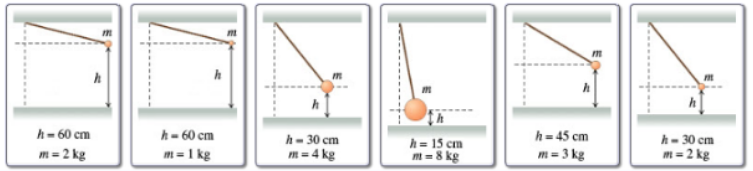# Problem: Rank each pendulum on the basis of the maximum kinetic energy it attains after release. Rank from largest to smallest.

###### FREE Expert Solution

Potential energy:

$\overline{){\mathbf{U}}{\mathbf{=}}{\mathbf{m}}{\mathbf{g}}{\mathbf{h}}}$

From the conservation of energy principle, Maximum kinetic energy is equal to maximum potential energy.

Take g = 10 m/s2

Label the pendulums as 1 to 6 from left to right.

Pendulum 1:

81% (55 ratings)###### Problem Details

Rank each pendulum on the basis of the maximum kinetic energy it attains after release.

Rank from largest to smallest.